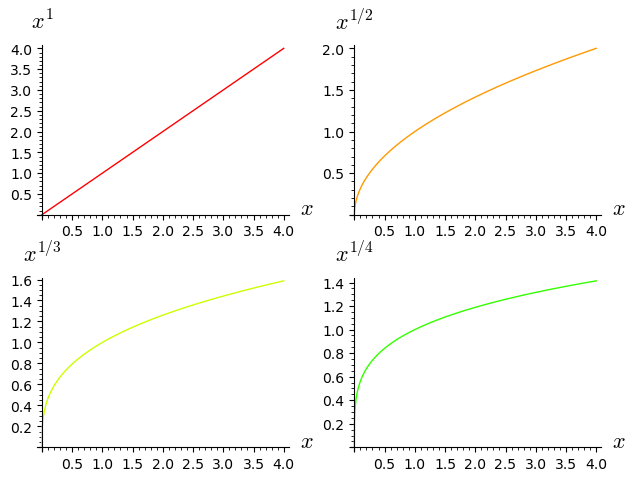# Evaluate variable in plot label

I have several plots with labels that I would like to evaluate. For instance

all=[plot(x^(1/(i+1)),(x,0,4), rgbcolor=hue(i/10),axes_labels=[r"$x$",r"$x^{1/(1+%d)}$"%i]) for i in range(4)]


leads to all showing me (sorry for not being able to upload due to <60 points) the plot lablel $x^{1/(1+1)}$, which I would like to display after evaluation ($x^{1/2}$)

By defining the function $f(i)=i+1$ in the preamble of the graph I still cannot evaluate $f$, for $i$ is not evaluated inside the list

Even supposing I would, suppose I want the exponent in digits form. I am unable to find the string splicing character which also evaluates and then displays 0.5 (instead of a single digit). Does anybody know about how to correct this syntax?

edit retag close merge delete

Sort by » oldest newest most voted

![sage: all=[plot(x^(1/(i+1)),(x,0,4), rgbcolor=hue(i/10),axes_labels=[r"$x$",r"$x^{%s}$"%str(1/(1+i))]) for i in range(4)]
sage: graphics_array(all, 2, 2)
Launched png viewer for Graphics Array of size 2 x 2]more